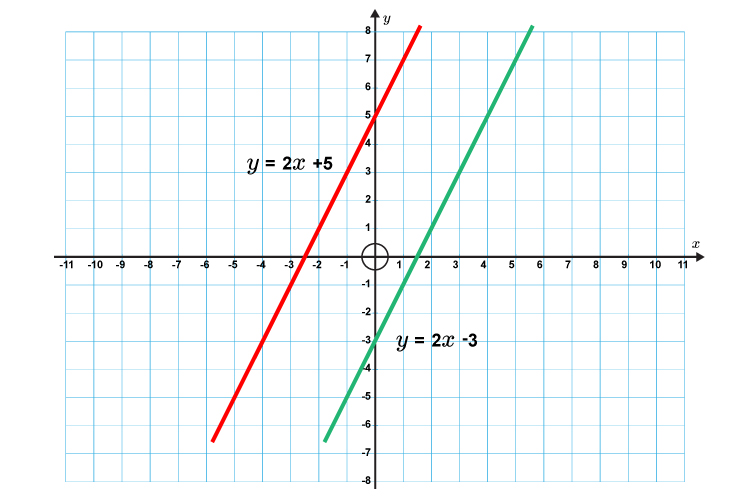# Parallel lines

Parallel lines have the same gradient (m)OF COURSE THEY DO

The formula for a straight line is  y=mx+c

The gradients of the lines above have the same value m.

In the example above the gradient is m = 2  on both straight line formulas.